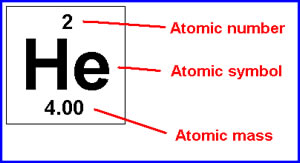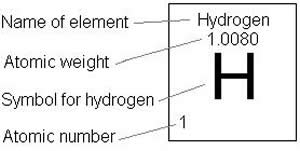# Difference between Atomic Mass and Atomic Weight

##### Key Difference: Atomic mass is defined as the total mass of protons, neutrons and electrons present in an atom of an element. Atomic weight is defined as the ratio of average mass of the atom present in an element. It is calculated to 1/12th of the mass of carbon atom. It is also referred to as relative atomic mass.

Both the terms, atomic mass and relative atomic mass read the same, but technically there is a big difference in the meaning of both the terms.Atomic mass is the mass of a single atomic particle or molecule. It is the sum of protons and electrons present in the atom of an element. It is expressed in mole. It is simply a collection of nuclides that make up a chemical element. The atomic mass can be calculated very precisely, as when the nuclides given in a specimen of an atom is exactly similar to any other given specimen, then the mass of these specimens is considered to be the same. Measurement and comparison of masses can be easily done with mass spectrometry. It is usually a whole number.Atomic weight is the ratio of atom of an element. The average weight of an atom is relative to the 1/12 weight of the carbon -12 atom. It is also referred to as relative atomic mass. Usually isotopes are included to calculate the average atomic weight. The value is not necessarily a whole number. The determination of atomic weight requires careful analysis of its compounds or given element during mass spectrometry. The mass of the abundant isotope contributes to the calculation of the atomic weight. For example for elements with two isotopes,

Atomic weight = Mass(a)*isotope(a)+ Mass(b)* isotope(b).

If Chlorine has two naturally-occurring isotopes where, Cl-35 mass is 34.968852 and isotope is 0.7577. Cl-37 mass is 36.965303 and isotope is 0.2423

atomic weight = massa x isotopea + massb x isotopeb

atomic weight = 34.968852 x 0.7577 + 36.965303 x 0.2423

atomic weight = 26.496 amu + 8.9566 amu

atomic weight = 35.45 amu

Basically, the presence of large number of isotopes in an element helps determine the difference between the atomic mass and atomic weight.

Comparison between Atomic mass and Atomic weight:

 Atomic Mass Atomic Weight Definition It is the sum of protons, neutrons and electrons present in an atom. It is the average ratio of atoms present in an element. Value Its is a whole number It is not necessarily a whole number. Isotopes These are usually not included in the calculation They are required for measuring the weight. Mass Mass of the given elements is 1. It is calculated to 1/12 ratio of a carbon atom. Analysis Based on mass spectrometry Based on mass spectrometry.

Image Courtesy: mistergunch.brinkster.net, homepages.ius.edu